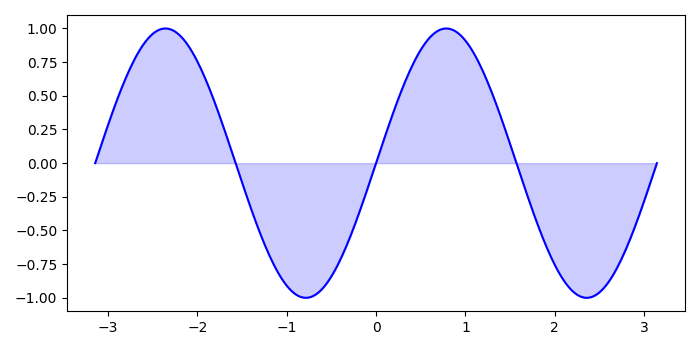# How to shade the regions between the curves in Matplotlib?

To shade the regions between curves, we can use the fill_between() method.

## Steps

• Initialize the variable n. Initiliize x and y data points using numpy.

• Create a figure and a set of subplots, fig and ax.

• Plot the curve using plot method.

• Use fill_between() method, fill the area between the two curves.

• To display the figure, use show() method.

## Example

import numpy as np
import matplotlib.pyplot as plt
plt.rcParams["figure.figsize"] = [7.00, 3.50]
plt.rcParams["figure.autolayout"] = True
n = 256
X = np.linspace(-np.pi, np.pi, n, endpoint=True)
Y = np.sin(2 * X)
fig, ax = plt.subplots()
ax.plot(X, Y, color='blue', alpha=1.0)
ax.fill_between(X, 0, Y, color='blue', alpha=.2)
plt.show()

## Output